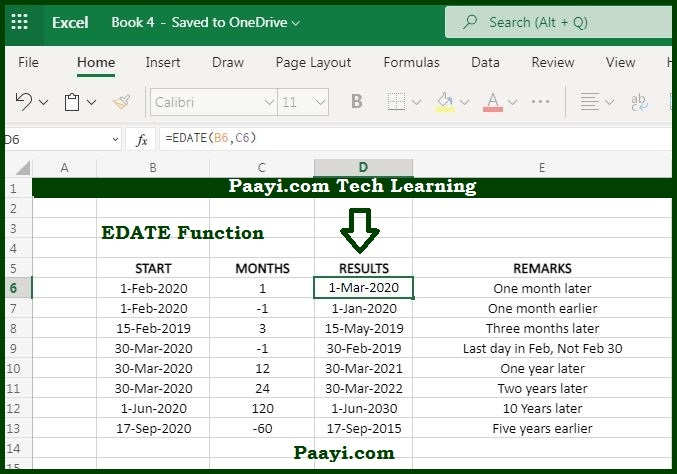# Learn How to Use Microsoft Excel EDATE Function

Written by | 0 Comments | 578 Views

In this article, you will learn how to use the Microsoft Excel EDATE function and its prime function in Microsoft Excel. You will also get to know the Microsoft Excel EDATE function return value and syntax with the help of some examples.

## Microsoft Excel EDATE Function

The purpose of the Microsoft Excel EDATE function is to shift dates and months in the future and past. That means the EDATE function returns the date on the same day of the month, n months in the past or future. The EDATE can be used to calculate expiration dates, mature dates, and other due dates. You need to use the positive value for months to get a date in the future and negative values to get dates in the past.

### EDATE Function Return Value

Return the new date as an Excel serial number.

### EDATE Function Syntax

= EDATE(start-date, months)

Where:

• start-date: The valid Excel start date.
• months: Number of months before or after the start specific date.

## How to Use Microsoft Excel EDATE Function?The EDATE function can add or subtract the months from the date. The various due dates can be calculated using the EDATE function of past and future. You need to use a positive number to get the future date and a negative number to get the past date. The EDATE function will return a serial number corresponding to the date. You can use the number format of your choice to display the result as a date.

Let's understand this by the example:

Example 1: Suppose A1 is having the date January 1, 2020, then you can use the EDATE functions as:

• =EDATE(A1, 1) - This will return the value on February 1, 2020.
• =EDATE(A1, 3) - This will return the value April 1, 2020.
• =EDATE(A1, -1) - This will return the value on December 1, 2019.
• =EDATE(A1, -3) - This will return the value October 1, 2019.

Example 2: To move the dates by years, you can use the EDATE function as given below; let's say A1 is having the date January 1, 2020.

• =EDATE(A1, 24) - This will return the two years future date.
• = EDATE(A1, -36) - This will return the three years past date.